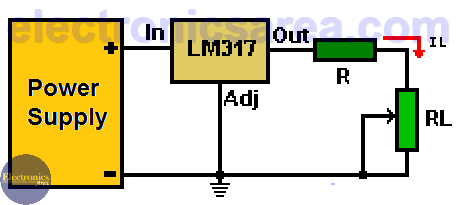# Variable Dummy Load for Power Supply Testing

It is easy to find on the Internet many designs of voltage sources, with which we can power our circuits designs. It would be very useful to know how to test a voltage source in order to determine if its characteristics are good or not. To achieve this goal we can use a variable dummy load, which in this case supports a maximum of 1.5 amps.

## The percentage regulation

The “percentage regulation” is a characteristic of the voltage source obtained with the formula:

Percentage regulation (%) = ([VNL – VFL] / VFL) x 100

where: VNL = No load output voltage, VFL = Full load output voltage.

The ideal characteristic of a voltage source (power supply) is to keep the output voltage constant no matter how much current the load requests.

Analyzing the above equation it can be concluded that this characteristic occurs when the percentage regulation is zero (“0”). (VNL – VFL = 0). Since voltage sources are real, not ideal, it is desirable that this percentage of regulation be as small as possible.## How to use a variable dummy load for power supply testing?

To implement a variable dummy load, a variable voltage regulator LM317 connected as shown in the following diagram is used. Due to its internal design, this voltage regulator has a voltage of 1.25 volts between its output terminals (Out) and adjustment terminals (Adj). So:

The voltage of 1.25 volts is applied to the R and RL in series resistors. Thus, the current IL flowing through the load resistor RL (the potentiometer) is: IL = 1.25 V / (R + RL).

Since RL is a variable resistor, the current passing through the load (RL) can be varied. In this way you can test the ability of the power source, to give a specific current for a specific voltage value, just by setting the current with the RL potentiometer.This test can be performed with sources with different output voltages (voltage that is applied to the input (In) of the voltage regulator).

Since RL is a variable resistor, its value could be “0” ohms, causing a short circuit. To avoid a short circuit, a series resistor R is used to protect the LM317 regulator. The RL and R values are chosen to obtain the minimum and maximum current with which you want to do the test. It is recommended that both R and RL be 2 watts or more.

Notes:

• Take into account that the maximum current that the LM317 can give is 1.5 amps. Also take into account the maximum current that can be supplied by the source to be tested, so as not to exceed it and damage it. If you want  the power supply to give more current, you have to use a circuit with higher capacity.
• (Very important) The voltage regulator must have a large heatsink, due to the large amount of current and voltage it could withstand. A computer fan can be placed to reduce the temperature, if necessary. A large microprocessor heater plus a corresponding fan can be used.
•  It would be convenient to place an ammeter in series with the resistor R.
• The tests must be done for limited period of time.
• 4
•
•
•
•
•
•
•
•
4
Shares
•
4
Shares
• 4
•
•
•
•
•
•
•
Tagged with: